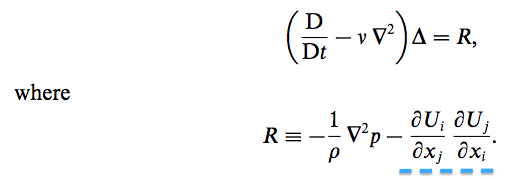# Divergence of the Navier-Stokes Equation

• I
The Navier-Stokes equation is:

(DUj/Dt) = v [(∂2Ui/∂xj∂xi) + (∂2Uj/∂xi∂xi)] – 1/ρ (∇p)

where D/Dt is the material (substantial) derivative, v is the kinematic viscosity and ∇p is the modified pressure gradient (taking into account gravity and pressure). Note that the velocity field is non-solenoidal (∇⋅U ≠ 0).

How, then, can we take the divergence of this equation and get the following result?:I can follow all of the terms other than the one underlined in blue. I know that it comes from the blue section of the Navier-Stokes written above, since I can easily get all the other terms.

Chestermiller
Mentor
The Navier-Stokes equation is:

(DUj/Dt) = v [(∂2Ui/∂xj∂xi) + (∂2Uj/∂xi∂xi)] – 1/ρ (∇p)

where D/Dt is the material (substantial) derivative, v is the kinematic viscosity and ∇p is the modified pressure gradient (taking into account gravity and pressure). Note that the velocity field is non-solenoidal (∇⋅U ≠ 0).

How, then, can we take the divergence of this equation and get the following result?:

View attachment 100112

I can follow all of the terms other than the one underlined in blue. I know that it comes from the blue section of the Navier-Stokes written above, since I can easily get all the other terms.